# Non Verbal Reasoning - Series

## Why Non Verbal Reasoning Series?

In this section you can learn and practice Non Verbal Reasoning Questions based on "Series" and improve your skills in order to face the interview, competitive examination and various entrance test (CAT, GATE, GRE, MAT, Bank Exam, Railway Exam etc.) with full confidence.

## Where can I get Non Verbal Reasoning Series questions and answers with explanation?

IndiaBIX provides you lots of fully solved Non Verbal Reasoning (Series) questions and answers with Explanation. Solved examples with detailed answer description, explanation are given and it would be easy to understand. All students, freshers can download Non Verbal Reasoning Series quiz questions with answers as PDF files and eBooks.

## Where can I get Non Verbal Reasoning Series Interview Questions and Answers (objective type, multiple choice)?

Here you can find objective type Non Verbal Reasoning Series questions and answers for interview and entrance examination. Multiple choice and true or false type questions are also provided.

## How to solve Non Verbal Reasoning Series problems?

You can easily solve all kind of Non Verbal Reasoning questions based on Series by practicing the objective type exercises given below, also get shortcut methods to solve Non Verbal Reasoning Series problems.

### Exercise :: Series - Section 1

Each of the following questions consists of five figures marked A, B, C, D and E called the Problem Figures followed by five other figures marked 1, 2, 3, 4 and 5 called the Answer Figures. Select a figure from amongst the Answer Figures which will continue the same series as established by the five Problem Figures.

1.

Select a figure from amongst the Answer Figures which will continue the same series as established by the five Problem Figures.

Problem Figures:                          Answer Figures: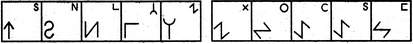(A)     (B)     (C)     (D)     (E)       (1)     (2)     (3)     (4)     (5)

 A. 1 B. 2 C. 3 D. 4 E. 5

Explanation:

In each step, element at the upper-right position gets enlarged, inverts vertically and reaches the lower-left corner; the existing element at the lower-left position, is lost and a new small element appears at the upper-right position.

2.

Select a figure from amongst the Answer Figures which will continue the same series as established by the five Problem Figures.

Problem Figures:                          Answer Figures: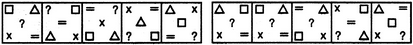(A)     (B)     (C)     (D)     (E)       (1)     (2)     (3)     (4)     (5)

 A. 1 B. 2 C. 3 D. 4 E. 5

Explanation:

In each step, the elements move in the sequence.

3.

Select a figure from amongst the Answer Figures which will continue the same series as established by the five Problem Figures.

Problem Figures:                          Answer Figures: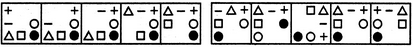(A)     (B)     (C)     (D)     (E)       (1)     (2)     (3)     (4)     (5)

 A. 1 B. 2 C. 3 D. 4 E. 5

Explanation:

In each step, the CW-end element moves to the ACW-end position.

4.

Select a figure from amongst the Answer Figures which will continue the same series as established by the five Problem Figures.

Problem Figures:                          Answer Figures: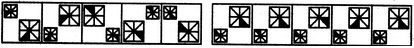(A)     (B)     (C)     (D)     (E)       (1)     (2)     (3)     (4)     (5)

 A. 1 B. 2 C. 3 D. 4 E. 5

Explanation:

Both the larger and the smaller squares move to the adjacent corner ACW in each turn. Also, the shading in the smaller square moves 1, 2, 3, 4, 5, ... steps ACW sequentially and the shading in the larger square moves 1, 2, 3, 4, 5,.... steps CW sequentially.

5.

Select a figure from amongst the Answer Figures which will continue the same series as established by the five Problem Figures.

Problem Figures:                          Answer Figures: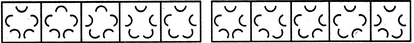(A)     (B)     (C)     (D)     (E)       (1)     (2)     (3)     (4)     (5)

 A. 1 B. 2 C. 3 D. 4 E. 5